﻿ 三角形网格下二维浅水方程的高分辨率格式

三角形网格下二维浅水方程的高分辨率格式A Non-Oscillatory Scheme for Shallow Water Equations on Triangular Meshes

Abstract: For the shallow water equations, a new finite volume NVSF (Normalized Variable and Space For-mulation) scheme is constructed under the triangular mesh. This scheme is based on the CBC (Convection Boundedness Criterion) criterion. NVSF scheme is adopted to solve the application of NVF high resolution scheme on triangular meshes, by comparing the exact solution of the typical example with the numerical solution. It is shown that the new numerical scheme has second order accuracy and has a good approximation near the exact solution. It can suppress the unphysical os-cillation of the discontinuity.

1. 引言

2. 非结构网格下新格式的构造

2.1. 非一致网格下NVSF的正则化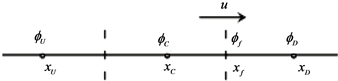Figure 1. Three neighboring mesh points and the mesh face

$\stackrel{˜}{\varphi }=\frac{\varphi -{\varphi }_{U}}{{\varphi }_{D}-{\varphi }_{U}}$ , $\stackrel{˜}{x}=\frac{x-{x}_{U}}{{x}_{D}-{x}_{U}}$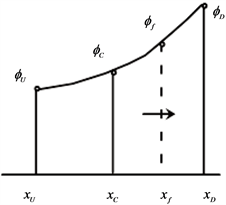Figure 2. The relationship between variables before the regularization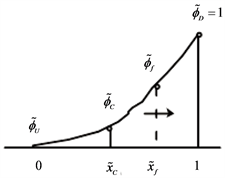Figure 3. The relationship between variables after the regularizationTable 1. The linear convection schemes on triangular meshes

2.2. 对流项有界性准则(1)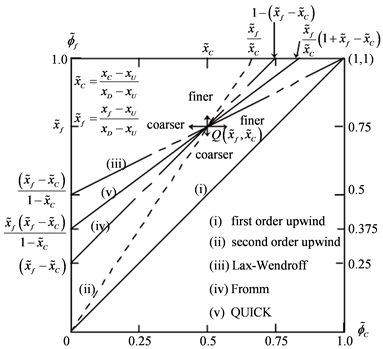Figure 4. Normalized Variable Diagram (NVD) for several linear schemes formulated using NVSF

2.3. 新格式的构造

${\stackrel{˜}{\varphi }}_{f}=a{\stackrel{˜}{\varphi }}_{C}^{4}+b{\stackrel{˜}{\varphi }}_{C}^{3}+c{\stackrel{˜}{\varphi }}_{C}^{2}+d{\stackrel{˜}{\varphi }}_{C}+e$ (2)

$\left\{\begin{array}{l}{\stackrel{˜}{\varphi }}_{f}\left(0\right)=0\\ {\stackrel{˜}{\varphi }}_{f}\left(1\right)=1\\ {\stackrel{˜}{\varphi }}_{f}\left({\stackrel{˜}{x}}_{C}\right)={\stackrel{˜}{x}}_{f}\\ {{\stackrel{˜}{\varphi }}^{\prime }}_{f}\left(1\right)=0\end{array}$ (3)

${{\stackrel{˜}{\varphi }}^{\prime }}_{f}\left({\stackrel{˜}{x}}_{C}\right)=\frac{{\stackrel{˜}{x}}_{f}\left({\stackrel{˜}{x}}_{f}-1\right)}{{\stackrel{˜}{x}}_{C}\left({\stackrel{˜}{x}}_{C}-1\right)}$ (4)

$\left\{\begin{array}{l}a=\frac{-\left({\stackrel{˜}{x}}_{C}^{3}-3{\stackrel{˜}{x}}_{C}^{2}+3{\stackrel{˜}{x}}_{C}{\stackrel{˜}{x}}_{f}-{\stackrel{˜}{x}}_{f}^{2}\right)}{{\stackrel{˜}{x}}_{C}^{2}{\left({\stackrel{˜}{x}}_{C}-1\right)}^{3}}\\ b=\frac{-\left(-2{\stackrel{˜}{x}}_{C}^{4}+4{\stackrel{˜}{x}}_{C}^{3}-4{\stackrel{˜}{x}}_{C}^{2}{\stackrel{˜}{x}}_{f}+4{\stackrel{˜}{x}}_{C}^{2}+{\stackrel{˜}{x}}_{C}{\stackrel{˜}{x}}_{f}^{2}-5{\stackrel{˜}{x}}_{C}{\stackrel{˜}{x}}_{f}+2{\stackrel{˜}{x}}_{f}^{2}\right)}{{\stackrel{˜}{x}}_{C}^{2}{\left({\stackrel{˜}{x}}_{C}-1\right)}^{3}}\\ c=\frac{-\left({\stackrel{˜}{x}}_{C}^{5}+{\stackrel{˜}{x}}_{C}^{4}-8{\stackrel{˜}{x}}_{C}^{3}+8{\stackrel{˜}{x}}_{C}^{2}{\stackrel{˜}{x}}_{f}-2{\stackrel{˜}{x}}_{C}{\stackrel{˜}{x}}_{f}^{2}+{\stackrel{˜}{x}}_{C}{\stackrel{˜}{x}}_{f}-{\stackrel{˜}{x}}_{f}^{2}\right)}{{\stackrel{˜}{x}}_{C}^{2}{\left({\stackrel{˜}{x}}_{C}-1\right)}^{3}}\\ d=\frac{-\left(-2{\stackrel{˜}{x}}_{C}^{4}+4{\stackrel{˜}{x}}_{C}^{3}-4{\stackrel{˜}{x}}_{C}{\stackrel{˜}{x}}_{f}+{\stackrel{˜}{x}}_{f}^{2}+{\stackrel{˜}{x}}_{f}\right)}{{\stackrel{˜}{x}}_{C}^{2}{\left({\stackrel{˜}{x}}_{C}-1\right)}^{3}}\\ e=0\end{array}$

$0<{\stackrel{˜}{\varphi }}_{C}<1$ 时，

$\begin{array}{c}{\stackrel{˜}{\varphi }}_{f}=\frac{-\left({\stackrel{˜}{x}}_{C}^{3}-3{\stackrel{˜}{x}}_{C}^{2}+3{\stackrel{˜}{x}}_{C}{\stackrel{˜}{x}}_{f}-{\stackrel{˜}{x}}_{f}^{2}\right)}{{\stackrel{˜}{x}}_{C}^{2}{\left({\stackrel{˜}{x}}_{C}-1\right)}^{3}}{\stackrel{˜}{\varphi }}_{C}^{4}\\ \text{\hspace{0.17em}}\text{\hspace{0.17em}}+\frac{-\left(-2{\stackrel{˜}{x}}_{C}^{4}+4{\stackrel{˜}{x}}_{C}^{3}-4{\stackrel{˜}{x}}_{C}^{2}{\stackrel{˜}{x}}_{f}+4{\stackrel{˜}{x}}_{C}^{2}+{\stackrel{˜}{x}}_{C}{\stackrel{˜}{x}}_{f}^{2}-5{\stackrel{˜}{x}}_{C}{\stackrel{˜}{x}}_{f}+2{\stackrel{˜}{x}}_{f}^{2}\right)}{{\stackrel{˜}{x}}_{C}^{2}{\left({\stackrel{˜}{x}}_{C}-1\right)}^{3}}{\stackrel{˜}{\varphi }}_{C}^{3}\\ \text{\hspace{0.17em}}\text{\hspace{0.17em}}+\frac{-\left({\stackrel{˜}{x}}_{C}^{5}+{\stackrel{˜}{x}}_{C}^{4}-8{\stackrel{˜}{x}}_{C}^{3}+8{\stackrel{˜}{x}}_{C}^{2}{\stackrel{˜}{x}}_{f}-2{\stackrel{˜}{x}}_{C}{\stackrel{˜}{x}}_{f}^{2}+{\stackrel{˜}{x}}_{C}{\stackrel{˜}{x}}_{f}-{\stackrel{˜}{x}}_{f}^{2}\right)}{{\stackrel{˜}{x}}_{C}^{2}{\left({\stackrel{˜}{x}}_{C}-1\right)}^{3}}{\stackrel{˜}{\varphi }}_{C}^{2}\\ \text{\hspace{0.17em}}\text{\hspace{0.17em}}+\frac{-\left(-2{\stackrel{˜}{x}}_{C}^{4}+4{\stackrel{˜}{x}}_{C}^{3}-4{\stackrel{˜}{x}}_{C}{\stackrel{˜}{x}}_{f}+{\stackrel{˜}{x}}_{f}^{2}+{\stackrel{˜}{x}}_{f}\right)}{{\stackrel{˜}{x}}_{C}^{2}{\left({\stackrel{˜}{x}}_{C}-1\right)}^{3}}{\stackrel{˜}{\varphi }}_{C}\end{array}$

${\stackrel{˜}{\varphi }}_{C}\le 0$ 或者 ${\stackrel{˜}{\varphi }}_{C}\ge 1$ 时， ${\stackrel{˜}{\varphi }}_{f}={\stackrel{˜}{\varphi }}_{C}$

$\left\{\begin{array}{l}{\stackrel{˜}{\varphi }}_{f}=-2{\stackrel{˜}{\varphi }}_{C}^{4}+5{\stackrel{˜}{\varphi }}_{C}^{3}-5{\stackrel{˜}{\varphi }}_{C}^{2}+3{\stackrel{˜}{\varphi }}_{C},\text{\hspace{0.17em}}\text{\hspace{0.17em}}\text{\hspace{0.17em}}\text{\hspace{0.17em}}\text{\hspace{0.17em}}\text{\hspace{0.17em}}\text{\hspace{0.17em}}0\le {\stackrel{˜}{\varphi }}_{C}\le 1\\ {\stackrel{˜}{\varphi }}_{f}=0,\text{\hspace{0.17em}}\text{\hspace{0.17em}}\text{\hspace{0.17em}}\text{\hspace{0.17em}}\text{\hspace{0.17em}}\text{\hspace{0.17em}}\text{\hspace{0.17em}}\text{\hspace{0.17em}}\text{\hspace{0.17em}}\text{\hspace{0.17em}}\text{\hspace{0.17em}}\text{\hspace{0.17em}}\text{\hspace{0.17em}}\text{\hspace{0.17em}}\text{\hspace{0.17em}}\text{\hspace{0.17em}}\text{\hspace{0.17em}}\text{\hspace{0.17em}}\text{\hspace{0.17em}}\text{\hspace{0.17em}}\text{\hspace{0.17em}}\text{\hspace{0.17em}}\text{\hspace{0.17em}}\text{\hspace{0.17em}}\text{\hspace{0.17em}}\text{\hspace{0.17em}}\text{\hspace{0.17em}}\text{\hspace{0.17em}}\text{\hspace{0.17em}}\text{\hspace{0.17em}}\text{\hspace{0.17em}}\text{\hspace{0.17em}}\text{\hspace{0.17em}}\text{\hspace{0.17em}}\text{\hspace{0.17em}}\text{\hspace{0.17em}}\text{elsewhere}\end{array}$ (5)

3. 二维浅水方程

$\frac{\partial U}{\partial t}+\nabla F=0$ (6)

U是守恒变量向量， $F=\left(E,G\right)$ ，E是x方向通量，G是y方向通量。其中

$U=\left(\begin{array}{c}h\\ hu\\ hv\end{array}\right)$ , $E=\left(\begin{array}{c}hu\\ h{u}^{2}+\frac{g{h}^{2}}{2}\\ huv\end{array}\right)$ , $G=\left(\begin{array}{c}hv\\ huv\\ h{v}^{2}+\frac{g{h}^{2}}{2}\end{array}\right)$

3.1. 有限体积法数值离散

${V}_{i}$ 上对(6)式积分可得：

${\int }_{{V}_{i}}\left(\frac{\partial U}{\partial t}+\nabla F\right)\text{d}V=0$

${\iint }_{A}\frac{\partial U}{\partial t}\text{d}A+{\iint }_{A}\nabla F\text{d}A=0$

${\iint }_{A}\left(\frac{\partial U}{\partial t}+\nabla F\right)\text{d}x\text{d}y=0$

$\frac{\partial }{\partial t}{\iint }_{A}U\text{d}A+{\iint }_{A}\nabla F\text{d}A=0$ (7)

${U}_{i}$ 为第i个单元控制体U上的平均值。

${U}_{i}=\frac{1}{{A}_{i}}{\iint }_{{A}_{i}}U\text{d}{A}_{i},\text{\hspace{0.17em}}\text{\hspace{0.17em}}\therefore {A}_{i}{U}_{i}={\iint }_{{A}_{i}}U\text{d}A$

$\frac{\partial {U}_{i}}{\partial t}=-\frac{1}{{A}_{i}}{\oint }_{\Gamma }Fn\text{d}\Gamma$ (8)

$n$$\Gamma$ 的单位外法向向量， $\Gamma$ 为控制单元体A的边界。

$\frac{\partial {U}_{i}}{\partial t}=-\frac{1}{{A}_{i}}\underset{j=1}{\overset{3}{\sum }}{F}_{ij}{n}_{ij}{l}_{ij}=-\frac{1}{{A}_{i}}\underset{j=1}{\overset{3}{\sum }}{F}_{ij}\Delta {l}_{ij}$

${n}_{ij}$ 是通过第i个单元控制体的第j条边上的单位外法向量；

${l}_{ij}$ 是第i个控制体的第j条边；

$\Delta {l}_{ij}$ 是第i个单元控制体的第j条边的长度。

${F}_{ij}^{Roe}=\frac{1}{2}\left[F\left({\varphi }_{ij}^{L}\right)+F\left({\varphi }_{ij}^{R}\right)-|{a}_{ij}|\left({\varphi }_{ij}^{R}-{\varphi }_{ij}^{L}\right)\right]$ , (9)

${a}_{ij}=\left\{\begin{array}{l}\frac{F\left({\varphi }_{ij}^{R}\right)-F\left({\varphi }_{ij}^{L}\right)}{{\varphi }_{ij}^{R}-{\varphi }_{ij}^{L}},\text{\hspace{0.17em}}\text{\hspace{0.17em}}\text{\hspace{0.17em}}{\varphi }^{L}\ne {\varphi }^{R}\\ {F}^{\prime }\left({\varphi }_{ij}^{L}\right),\text{\hspace{0.17em}}\text{\hspace{0.17em}}\text{\hspace{0.17em}}\text{\hspace{0.17em}}\text{\hspace{0.17em}}\text{\hspace{0.17em}}\text{\hspace{0.17em}}\text{\hspace{0.17em}}\text{\hspace{0.17em}}\text{\hspace{0.17em}}\text{\hspace{0.17em}}\text{\hspace{0.17em}}\text{\hspace{0.17em}}\text{\hspace{0.17em}}\text{ }{\varphi }^{L}={\varphi }^{R}\end{array}$ (10)

4. 时间的离散

$\left\{\begin{array}{l}{\varphi }^{\left(1\right)}={\varphi }^{n}+L\left({\varphi }^{\left(1\right)}\right)\\ {\varphi }^{\left(2\right)}=\frac{3}{4}{\varphi }^{n}+\frac{2}{3}\left({\varphi }^{\left(1\right)}+L\left({\varphi }^{\left(1\right)}\right)\right)\\ {\varphi }^{\left(n+1\right)}=\frac{1}{3}{\varphi }^{n}+\frac{2}{3}\left({\varphi }^{\left(n\right)}+L\left({\varphi }^{\left(n\right)}\right)\right)\end{array}$ (11)

5. 数值算例

5.1. 一维线性对流方程

$\left\{\begin{array}{l}\frac{\partial \varphi }{\partial t}+a\frac{\partial \varphi }{\partial x}=0,\text{\hspace{0.17em}}\text{\hspace{0.17em}}x\in \left[a,b\right],t>0\\ \varphi \left(x,0\right)={\varphi }_{0}\left(x\right)\end{array}$ (12)

5.1.1. 情形1

${‖E‖}_{p}={\left(\Delta x\underset{i=1}{\overset{N}{\sum }}|{\stackrel{¯}{\phi }}_{i}\left(\text{exact}\right)-{\stackrel{¯}{\phi }}_{i}\left(\text{computed}\right)|\right)}^{\frac{1}{p}},\text{\hspace{0.17em}}p=1,2$

$\text{order}=\frac{\mathrm{ln}\frac{{E}_{N}}{{E}_{2N}}}{\mathrm{ln}2}$Table 2. Errors and orders for several selected schemes

5.1.2. 情形2

$\varphi \left(x,0\right)=\left\{\begin{array}{l}1,x\le 0\\ 0,x\ge 0\end{array}$

5.1.3. 情形3

$\varphi \left(x,0\right)=\left\{\begin{array}{l}1,\text{\hspace{0.17em}}\text{\hspace{0.17em}}\text{\hspace{0.17em}}\text{\hspace{0.17em}}\text{\hspace{0.17em}}\text{\hspace{0.17em}}\text{\hspace{0.17em}}\text{\hspace{0.17em}}\text{\hspace{0.17em}}\text{\hspace{0.17em}}\text{\hspace{0.17em}}\text{\hspace{0.17em}}\text{\hspace{0.17em}}\text{\hspace{0.17em}}\text{\hspace{0.17em}}\text{\hspace{0.17em}}\text{\hspace{0.17em}}0\le x\le 0.2\\ 4x-0.6,\text{\hspace{0.17em}}\text{\hspace{0.17em}}\text{\hspace{0.17em}}\text{\hspace{0.17em}}\text{\hspace{0.17em}}\text{\hspace{0.17em}}0.2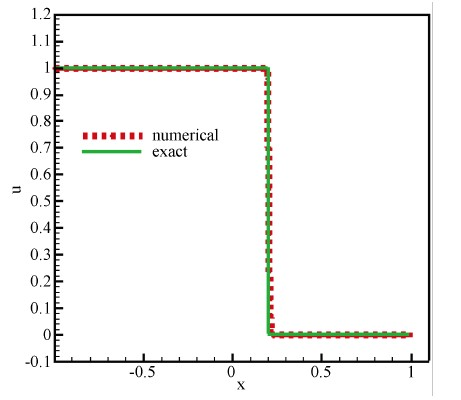Figure 5. Comparison of numerical and exact results for the new condition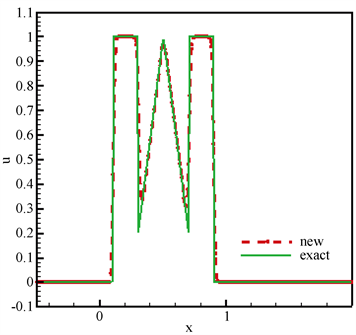Figure 6. Comparison of numerical and exact results for the non-smooth initial condition

5.2. 二维线性对流方程

$\frac{\partial U}{\partial t}+\frac{\partial E}{\partial x}+\frac{\partial G}{\partial y}=0$

U是守恒变量向量，E是x方向通量，G是y方向通量。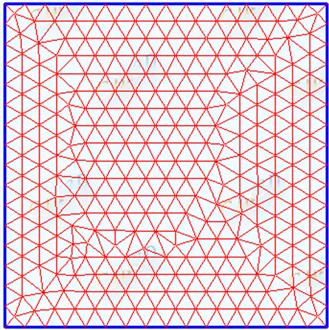Figure 7. Diagram of triangulation of Easy Mesh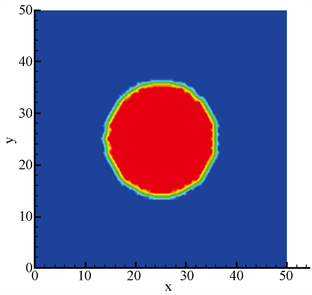(a)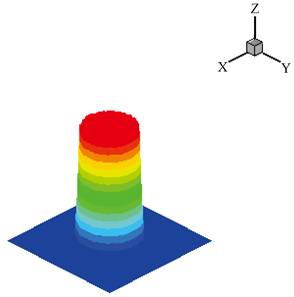(b)

Figure 8. (a) 2D exact solutions of shallow water equations; (b) 3D exact solutions of shallow water equations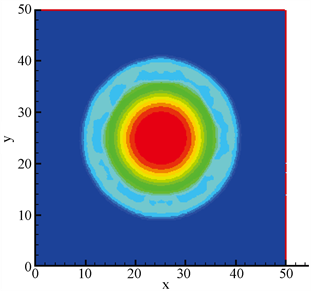(a)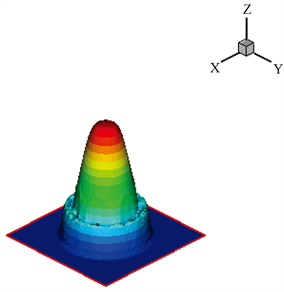(b)

Figure 9. (a) 2D numerical solutions of shallow water equations; (b) 3D numerical solutions of shallow water equations

6. 结论

 郭彦, 基于特征思想的高分辨率格式的研究与应用[D]: [博士学位论文]. 合肥: 中国科学技术大学. 2009.

 王立辉, 胡四一, 龚春生. 二维浅水方程的非结构网格数值解[J]. 水利水运工程学报, 2006, 13(1): 8-13.

 窦红, 汪继文. 求解二维浅水方程的一种高分辨率有限体积格式[J]. 应用数学和计算数学学报, 2006, 20(2): 83-88.

 王昆, 金生, 髙述峰, 宋立娜, 哈斯. 基于非结构网格的Godunov格式的二维浅水有限体积数值计算模式[J]. 中国水运, 2008, 8(5): 97-98.

 朱华君, 宋松和. 二维浅水波方程的非结构网格ENO型有限体积法[J]. 湖南师范大学自然科学报, 2007, 30(1): 21-26.

 Spalding, D.B. (1972) A Novel Finite Difference Formulation for Differential Expressions Involving Both First and Second Derivatives. International Journal for Numerical Methods in Engineering, 4, 551-559.
https://doi.org/10.1002/nme.1620040409

 Lenard, B.P. (1988) Simple High-Accuracy Resolution Program for Convective Modeling of Discontinuities. International Journal for Numerical Methods in Fluids, 8, 1291-1318.
https://doi.org/10.1002/fld.1650081013

 Wei, J.J., Yu, B.., Tao, W.Q., Kawaguchi, Y. and Wang, H.S. (2003) A New High-Order-Accurate and Bounded Scheme for Incompressible Flow. Numerical Heat Transfer, Part B: Fundamentals, 43, 19-41.
https://doi.org/10.1080/713836153

 Gaskell, P.H. and Lau, A.K.C. (1988) Curvature-Compensated Convective Transport: SMART, A New Roundedness-Preserving Transport Algorithm. International Journal for Numerical Methods in Fluids, 8, 617-641.
https://doi.org/10.1002/fld.1650080602

 Chakravarthy, S.R. and Osher, S. (1983) High Resolution Applications of the OSHER Upwind Scheme for the Euler Equations. AIAA Paper 83-1943.
https://doi.org/10.2514/6.1983-1943

 Darwish, M.S. and Moukallod, F.H. (1994) Normalized Variable and Space Formulation Methodology for High-Resolution Schemes. Numerical Heat Transfer, Part B, 26, 79-96.
https://doi.org/10.1080/10407799408914918

 Spalding, D.B. (1972) A Novel Finite Difference Formulation for Differential Expressions Involving Both First and Second Derivatives. International Journal for Numerical Methods in Engineering, 4, 551-559.
https://doi.org/10.1002/nme.1620040409

 Lenard, B.P. (1988) Simple High-Accuracy Resolution Program for Convective Modeling of Discontinuities. International Journal for Numerical Methods in Fluids, 8, 1291-1318.
https://doi.org/10.1002/fld.1650081013

 Wei, J.J., Yu, B., Tao, W.Q., Kawaguchi, Y. and Wang, H.S. (2003) A New High-Order-Accurate and Bounded Scheme for Incompressible Flow. Numerical Heat Transfer, Part B: Fundamentals, 43, 19-41.
https://doi.org/10.1080/713836153

 Van Leer, B. (1977) Towards the Ultimate Conservative Difference Scheme. V. A Second-Order Sequel to Godunov's Method. Journal of Computational Physics, 23, 101-136.
https://doi.org/10.1016/0021-9991(77)90095-X

 Roe, P.L. (1980) Approximate Riemann Solvers, Parameter Vectors and Difference Schemes. Journal of Computational Physics, 43, 357-372.
https://doi.org/10.1016/0021-9991(81)90128-5

 Gottlieb, S. and Shu, C.-W. (1998) Total Variational Diminishing Runge-Kutta Schemes. Mathematics of Computation, 67, 73-85.
https://doi.org/10.1090/S0025-5718-98-00913-2

Top